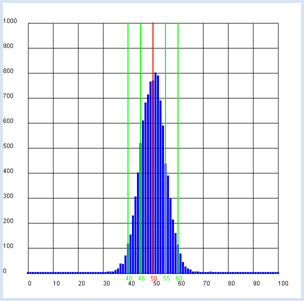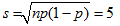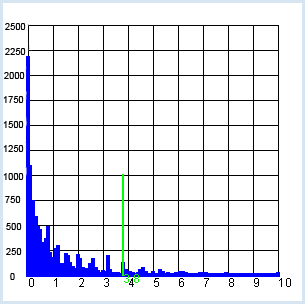deutsch     english    français     Print

## 8.3 HYPOTHESES, STATISTICAL TESTS### INTRODUCTION

 You make a hypothesis (called the null hypothesis), for example to check if the coin lying in front of you is not a fake, which means that the probability for landing on heads and tails is the same (p = ½). Or, you might have a die in front of you and make a hypothesis that it is not loaded, which means that all 6 numbers have the same probability of occurring (p = 1/6). In this chapter you will learn a method to test your hypothesis, however not with absolute certainty as you assume a 5% probability (significance level) with which the null hypothesis is wrongly rejected. PROGRAMMING CONCEPTS: Null hypothesis, significance, dispersion, Chi-square test

### A SIGNIFICANTLY FAKE COIN

You begin with the null hypothesis that the coin is not a fake and if you toss it n = 100 times, you get heads a certain number of times k and tails n - k times.

 You repeat the test several times, let's say z = 10,000 times, and the result is a distribution for k that you can determine with a simulation. As you expect, it is in a bell-shaped distribution around the average value m = 50 [more...It is a binomial distribution, which can be approximated by a normal distribution]. You now take on the interesting question of in which area +- s around the average value  a predetermined percentage lays, e.g. 68 % of all tests. You can also determine s, called dispersion, in the computer simulation by adding up the frequencies to the left and right starting at the average until you reach 6800.If you mark the area corresponding to 95% of all cases, you obtain approximately double the dispersion (here between 40 and 60).

```from gpanel import *
from random import random

n = 100 # size of the test  group
p = 0.5
z = 10000

def showDistribution():
setColor("blue")
lineWidth(4)
for t in range(n + 1):
line(t, 0, t, h[t])

def showMean():
global mean
count = 0
for t in range(n + 1):
count += h[t] * t
mean = int(count / z + 0.5)
setColor("red")
lineWidth(2)
line(mean, 0, mean, 1000)
text(mean - 1, -30, str(mean))

count = h[mean]
for s in range(1, 20):
count += h[mean + s] + h[mean - s]
if count > z * level:
break
setColor("green")
lineWidth(2)
line(mean + s, 0, mean + s, 1000)
text(mean + s - 1, -30, str(mean + s))
line(mean - s, 0, mean - s, 1000)
text(mean - s - 1, -30, str(mean - s))

def sim():
count = 0
repeat n:
w = random()
if w < p:
count +=1
return count

makeGPanel(-0.1 * n, 1.1 * n, -100, 1100)
title("Coin toss,  distribution of number")
drawGrid(0, n, 0, 1000)
h =  * (n + 1)

repeat z:
k = sim()
h[k] += 1

showDistribution()
showMean()
```
Programmcode markieren (Ctrl+C kopieren, Ctrl+V einfügen)

### MEMO

 If you frequently make a test with 100 coins that are not fake, in 68 % of all cases the number of tossed heads lies in the area 50 +-5, and 95% of all cases in the area 50 +-10 [more... The theoretically calculated value is] . If you make a test with the coin that is lying in front of you and you get a value for the number of heads that is greater than 60 or smaller than 40 you reject the hypothesis that the coin is not fake, in other words, you say that the coin is fake. In this case, you may be mistaken with a probability of 5% (the significance level). Sometimes you can also concisely say that the present coin is significantly fake.

###You have a die in front of you and want to test whether it is a fair die, which means that all numbers can occur with the same probability of 1/6. You make the hypothesis: The die is not loaded.

Here you will get to know a slightly different method from one that we used with the coin since there are six, not only two, possibilities that can occur on a roll, namely the numbers from 1 to 6. To be on the safe side you will want to roll the die often, let's say around 600 times, and write down the frequencies of the numbers that occur.

 Pip number Observed frequency (u) Theoretical frequency (expected value e) 1 112 100 2 128 100 3 97 100 4 103 100 5 88 100 6 72 100 Total 600 600

Observed and theoretical frequencies

In order to introduce a measure for the deviation of the observed from the theoretical occurrences, you need to calculate the relative square deviation for each number (u - e)2 / eand add up these values. We call the result χ2 (pronounced "Chi-square").

 This raises the interesting question of how χ2is distributed, meaning how often the different values of χ2 occur in many 600-roll attempts. To find this out, perform another computer simulation with 10,000 samples and determine the distribution. For the sake of simplicity, you can round the obtained values to whole numbers [more... The result is the famous χ2 distribution for the degree of freedom 6 - 1 = 5]. Coincidentally, you again enter a critical value for χ2, below 95% of all cases. The simulation results in s = 11 [more... this is the value obtained from a table for the χ2 distribution in one degree of freedom and a significance of 5 of 0.95].```from gpanel import *
from random import random, randint

n = 600 # number of tosses
p = 1 / 6
z = 10000

def showDistribution():
setColor("blue")
lineWidth(4)
for i in range(21):
line(i, 0, i, h[i])

def showLimit(level):
count = 0
for i in range(21):
count += h[i]
if count > z * level:
break
setColor("green")
lineWidth(2)
line(i, 0, i, 2000)
text(i, -80, str(i))
return i

def chisquare(u):
chi = 0
e = n * p
for i in range(1, 7):
chi += ((u[i] - e) * (u[i] - e)) / e
return chi

def sim():
u =  * 7
repeat n:
t = randint(1, 6)
u[t] += 1
return chisquare(u)

makeGPanel(-2, 22, -200, 2200)
title("Chi-square simulation  is being carried out. Please wait...")
drawGrid(0, 20, 0, 2000)
h =  * 21

repeat z:
c = int(sim())
if c < 20:
h[c] += 1
else:
h += 1

title("Chi-square test on  the die")
showDistribution()
s = showLimit(0.95)

# Observed series
u1 = [0, 112, 128, 97, 103, 88, 72]
u2 = [0, 112, 108, 97, 113, 88, 82]
c1 = chisquare(u1)
c2 = chisquare(u2)
print "Die with", u1, "Xi-square:", c1, "loaded?", c1 > s
print "Die with", u2, "Xi-square:", c2, "loaded?", c2 > s
```
Highlight program code (Ctrl+C to copy, Ctrl+V to paste)

### MEMO

 The computer simulation exposes the following result: in 95% of all cases, χ2 is less than or equal to the critical value 11. Hence, you have found a method to test if your die is rigged: calculate χ2 from the observed frequency. If the value is greater than 11, you can say with a 5% probability of being wrong that your null hypothesis of it being a fair die is incorrect, and therefore the die is loaded. The frequencies of the table above result in χ2 = 18.7. In other words, the die has a very high probability of being loaded. With another die rolled 600 times you get the frequencies u2 = [112, 108, 97, 113, 88, 82]. Since you obtain χ2 = 8.5, there is a low probability that the die is loaded.

### DIFFERENCES IN HUMAN BEHAVIOR

You can also apply the χ2 test to a study of the behavior of two groups of people. An interesting question often asked is whether in a particular context the behavior of females and males should be appraised to be statistically different, or whether both sexes behave equally.

You assume that the use of Facebook is studied in a secondary school. A total of 106 girls (women) and 86 boys (men) were asked whether they have a Facebook account. The survey results are as follows:

 Facebook Yes Facebook No Total % Yes Females 87 19 106 82.0% Males 62 24 86 72.1% Total 149 43 192 77.7%

The percentage of people who have a Facebook account is substantially greater among females than it is with males. But it raises the question of whether this higher proportion is statistically significant.

 For the simulation, you first determine the probability p of having an account from the total number n of females and males: p = (females_yes + males_yes) / n With this value you simulate the number of females who have an account using random numbers and the total number of females. This results in f0 females with an account and f1 females without one. You do the same for the males, and you will get m0 males with an account and m1 men without one. These numbers form the values u in the calculation of  χ2. χ 2 = sum of (u - e)2 / eYou must now still determine the expected value e for all four cases. You can assume that p = (f0 + m0) / n is the total probability for a Yes and correspondingly 1 - p is the total probability for a No, so you calculate:

 Expected value for females- Yes: ef0 = total number of females * p Expected value for males- Yes: em0 = total number of males * p Expected value for females- No: ef1 = total number of females * (1 - p) Expected value for men- No em1 = total number of males * (1 - p)

The rest of the program remains largely unchanged from the die test.

```from gpanel import *
from random import random

z = 10000
# survey values/polls
females_yes = 87
females_no = 19
males_yes = 62
males_no = 24

def showDistribution():
setColor("blue")
lineWidth(4)
for i in range(101):
line(i/10, 0, i/10, h[i])

def showLimit(level):
count = 0
for i in range(101):
count += h[i]
if count > level * z:
break
setColor("green")
lineWidth(2)
limit = i / 10
line(limit, 0, limit, 1000)
text(limit, -80, str(limit))
return limit

def chisquare(f0, f1, m0, m1):
# f: females, m: males, 0:yes, 1:no
w = (f0 + m0) / n # probability of a yes
# expected value
ef0 = (f0 + f1) * w # females-yes
em0 = (m0 + m1) * w # males-yes
ef1 = (f0 + f1) * (1 - w) # females-no
em1 = (m0 + m1) * (1 - w) # males-no
# add up deviations (u - e)*(u - e) / e
chi = (f0 - ef0) * (f0 - ef0) / ef0 \
+ (m0 - em0) * (m0 - em0) / em0 \
+ (f1 - ef1) * (f1 - ef1) / ef1 \
+ (m1 - em1) * (m1 - em1) / em1
return chi

def sim():
# simulate females
f0 = 0 # yes
f1 = 0 # no
for i in range(females_all):
t = random()
if t < p:
f0 += 1
else:
f1 += 1
# simulate males
m0 = 0 # yes
m1 = 1 # no
for i in range(males_all):
t = random()
if t < p:
m0 += 1
else:
m1 += 1
return chisquare(f0, f1, m0, m1)

females_all = females_yes + females_no
males_all = males_yes + males_no
n = females_all + males_all  # all
p = (females_yes + males_yes) / n  # probability of yes for all
print "Facebook yes (all):", round(100 * p, 1), "%"
pf = females_yes / females_all
print "Facebook yes (females):", round(100 * pf, 1), "%"
pm = males_yes / males_all
print "Facebook yes (males:)", round(100 * pm, 1), "%"

makeGPanel(-1, 11, -250, 2750)
drawGrid(0, 10, 0, 2500)
h =  * 101

repeat z:
c = int(10 * sim())  # magnification factor of 10
if c < 100:
h[c] += 1
else:
h += 1

showDistribution()
s = showLimit(0.95)

c = chisquare(females_yes, females_no, males_yes, males_no)
print "critical value:", s
print "observed:", c,
if c <= s:
print "- the same behavior"
else:
print "- not the same behavior"
```
Highlight program code (Ctrl+C to copy, Ctrl+V to paste)

### MEMO

 The result is astonishing: the χ2significance limit is 3.8 [more... The value corresponds to the value of the χ2 table for 1 degree of freedom a significance of 0.95]. The survey values resulted in the smaller value of 2.7. Even though the proportion of females with accounts is essentially higher, it cannot be statistically proven that they differ substantially from the males with respect to Facebook.

### EXERCISES

1.

A classic roulette table has 37 numbers from 0 to 36 that should occur with equal probability. A clever player wants to detect some irregularities in order to increase their chance of winning. They make notes of the frequency of the numbers that occur in 1,000 games and get:

u = [20, 26, 20, 22, 20, 27, 18, 28, 21, 36, 20, 28, 25, 19, 22, 25, 33, 25, 28, 25, 32, 29, 22, 32, 28, 31, 26, 25, 32, 32, 25, 20, 25, 44, 40, 24, 45]

Use a χ2 test to check the null hypothesis that the roulette is fair.

2.

In order to scientifically test a medication, it is prescribed in a blind study to two groups of sick people, where one of the groups receives a placebo. The following values were found after the treatment:

 After treatment- cured After treatment- sick %of people cured Treated with medication 22 13 62.9 % Treated with placebo 11 17 39.3 %

The proportion of people cured with medical therapy is much greater than those without. Can we assume that the medication is effective?